Courses

# Doc- Review: Sequence & Series of complex numbers Mathematics Notes | EduRev

## Mathematics : Doc- Review: Sequence & Series of complex numbers Mathematics Notes | EduRev

The document Doc- Review: Sequence & Series of complex numbers Mathematics Notes | EduRev is a part of the Mathematics Course Topic-wise Tests & Solved Examples for IIT JAM Mathematics.
All you need of Mathematics at this link: Mathematics

Review
Sequence & Series of complex numbers
First consider the useful facts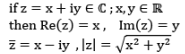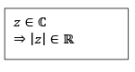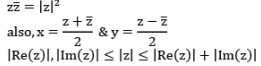The △ in-equality : If z1 & z2 are arbitrary complex no., then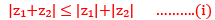Proof: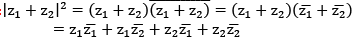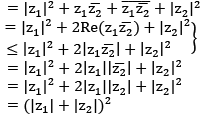Taking positive sq. root yields the desired inequality.

Note: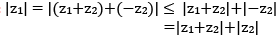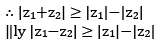Note equality occur in (i)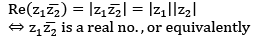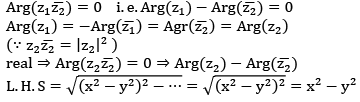Note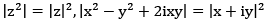Defn A seq. {zn} of complex no. is said to converge to a complex no. z if the seq. {|zn- z|} of real no. converge to 0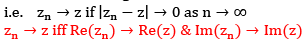Proof forward Part :
Assume that zn → z then |zn- z|→ 0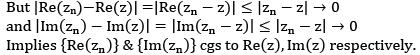Converse Part : Assume that Re(zn) → Re(z)  &  Im(zn) → Im(z)

consider,

|zn -  z|= |(xn + iyn )- (x+ iy)| where, zn = xn + iyn  & z = x + iy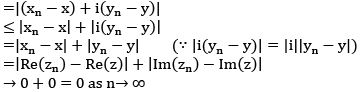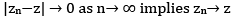Note: {zn} can’t converge to more than one limit.
If exists is unique.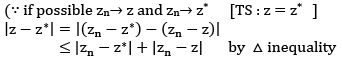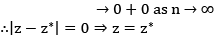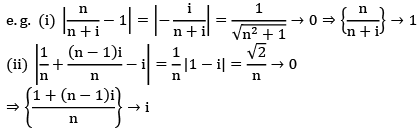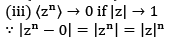→ 0 as n→∞ (∵0 ≤|z|≤ 1)

Defn A seq. {zn} of complex no. is called a Cauchy seq. if for each 𝜖 > 0, there exists (N=N(𝜖)) an integer N s.t.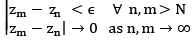Cauchy Criteria for convergence in complex plane {zn} converges iff {zn} is a Cauchy seq.

Forward part: Assume that zn → z then

Re(zn) → Re(z)   & Im(zn) → Im(z)

Proved before
Here {Re(zn)} & {Im(zn)} both are Cauchy seq. being convergent seq. of real no.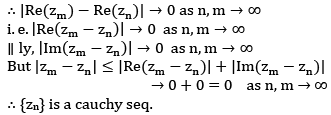Converse part: suppose {zm} is a Cauchy seq. , then using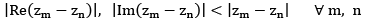implies both {Re(zn) & Im(zn)} are cauchy seq. of real no. hence both converges,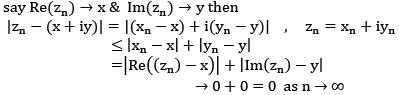implies {zn} converges
Defn The infinite series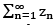of complex nos. is said to be converge if the seq. {Sn},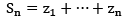of paritial sum S is convergent.

from the Cauchy criterion, i.e. “a seq. is conv. iff it is a Cauchy seq”, we see that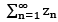converges iff {Sn}is a cauchy seq.

For each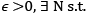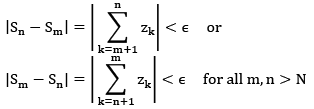from this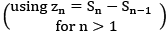it follows that convergence of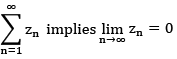(i. e. a neccessary cond for the series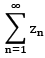to converge is that zn →0  as n→ ∞

Remark's in the case of sequence we have,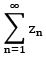converges to z iff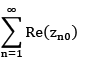converges to Re(z)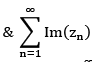converge to Im(z).
A sufficient condn for cgces of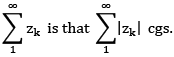i.e. absolutely convergent series is cgt.

also, as with real series, we say a series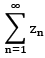is said to be absolutely convergent if the series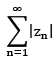+ve real no. is convergent. Futher using the fact that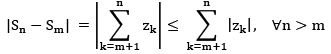Implies that “every absolutely convergent series is convergent.” Converse is not true e.g.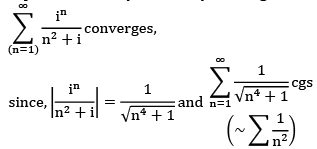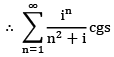absolutely and hence cgt.

Generalized Cauchy’s nth root test: let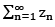be a series of complex term such that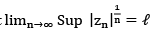(a) if ℓ < 1 then series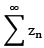cgs abs.
(b) if ℓ >1 then series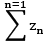div.

(c) if  ℓ = 1 the series may or may not conv.

Generalised D’ lembert Ratio test: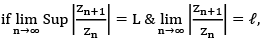then
(a) if L < 1, then the series cgs absolutely
(b) if l > 1, the series div.
(c)if l≤1≤ L, no conclusion.

complex analysis ( Bak &  Newmann)

Topology of the complex plane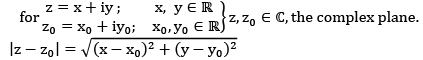1.5 Definition
(i)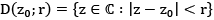is called an open disc of radius r centered at z0 , also called nbhd of z0.

(ii) circle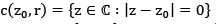= circle with center z0 , radius r.

(iii) subset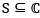is called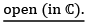If for every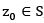, there exists r > 0 . It means that some disc arround z lies entirely in S. for instance, the interior of a circle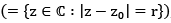the entire complex plane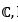half plane (Re(z) , Im(z) <0 , Re(z) ) ect. are open sets. An open disc is an open set.
(iv) A set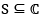is s. t. b open iff for each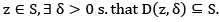Note: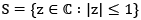is not open

Note: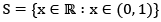is open in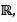but not in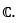(v) set S is called closed if its complement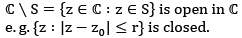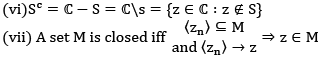= Coll of points whose neighbourhood have a non empty intersection with both S and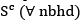(ix)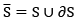= closure of S

(x) S is bdry iff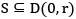for some r > 0.
(xi) S is compact iff S is closed bdd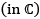(xii) set S is said to be disconneced if there exists two disjoint open set s. t.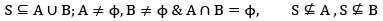(xiii) S is s. t. b. disconnected iff S is a union of two non empty disjoit open subsets.
(xiv) S is called connected if it is not disconnected. in other words, S is connected if and only if, each pair of points z1, z1 of S can be connected by an arc lying in S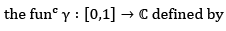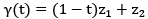is calle the line segment with and points z1 & z2 and denoted by [z1,z2]

∴ if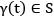for each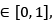then the line segment [z1, z2] , (where z1 , z2 ∈S ) is said to be containe in S.
→ by a polygonal line from z1 to zn … . a finite union of line segments of the form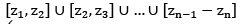(z1 and zn are said to be polygonally connected)
→ A set S is said to be polygonally connected if any two points of S can be connected by a polygonal line (basically horizonal or verticaly) contained in S.

Offer running on EduRev: Apply code STAYHOME200 to get INR 200 off on our premium plan EduRev Infinity!

## Topic-wise Tests & Solved Examples for IIT JAM Mathematics

27 docs|150 tests

,

,

,

,

,

,

,

,

,

,

,

,

,

,

,

,

,

,

,

,

,

;